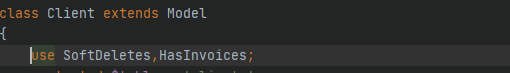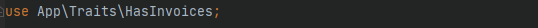﻿ laravel下trait的使用代码实例详解_php实例_脚本之家
php实例# laravel下trait的使用代码实例详解

Trait是PHP5.4引入的新概念，定义方式和class的定义方式类似。但是并不具备class的完整性。Trait看上去更像是一个class的一部分。它使不相关的两个class能够具有类似的行为。

Trait的简单使用Trait的引入

Trait的引入十分简单，在需要代码块的class中使用use Trait名就行。```<?php
class A{
public \$action = "逛街";
public \$money = 200;
public function action(){
echo "花了".\$this->money.\$this->action."\n";
}
}

class B{
public \$action = "吃骨头";
public \$time = 2;
public function action(){
echo "花了".\$this->time."分钟".\$this->action."\n";
}
}

class C{
public \$action = "跳舞";
public \$time = 30;
public function action(){
echo "花了".\$this->time."分钟".\$this->action."\n";
}
}
\$a = new A;
\$b = new B;
\$c = new C;
echo \$a->action();
echo \$b->action();
echo \$c->action();``````trait D{
public function action(){
echo "花了".(property_exists(\$this,'time')? \$this->time."分钟":\$this->money)
.\$this->action."\n";
}
}

class A{
use D;
public \$action = "逛街";
public \$money = 200;
}

class B{
use D;
public \$action = "吃骨头";
public \$time = 2;
}

class C{
use D;
public \$action = "跳舞";
public \$time = 30;
}
\$a = new A;
\$b = new B;
\$c = new C;
echo \$a->action();
echo \$b->action();
echo \$c->action();```